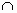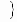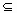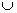Sets and Logical Connections

Sets are groups of certain, definably different elements (objects). In the 19th century, Danish mathematician Georg Cantor founded the study of sets, which studies most relations between individual sets.

A mathematical example of this is the set of natural numbers, N = {1,2,3,…}, which is part of the set of whole numbers Z = { …, -3,-2,-1,0,1,2,3,…} . We say, therefore, that the set of natural numbers is a subset of the set of whole numbers.

However, the study of sets is applicable to concrete objects. For example, a set of people wearing jeans is a subset of people who wear pants. Or the set of camembert cheeses is a subset of all kinds of cheeses.

Objects of one set are called elements. If an object x is an element of some set,

we write: xÎ M

and say that "x is an element of the set of M".

Some standardly used symbols are:

N = the set of natural numbers
Z = the set of whole numbers
Q = the set of rational numbers
R = the set of real numbers
Î = is an element…
Ï = is not an element…
Æ , { } = an empty set
È = unification of sets
Ç = intersection of sets
Í = is a subset of / is contained in…
Ì = is a true subset of / is entirely contained in
If we want to defined a certain set, we write
M =
{ … }

All elements which belong to M are written in the brackets. Therefore, a set is defined by a list and the individual elements are separated by a comma. For example:

M = { …, -2, -1, 0, 1, 2, … } is a set of all whole numbers
M =
{ …, Eidam, Camembert, Gouda, Brie, Parenica,…} is a set of all cheeses
M =
{ 2, 3, 5, 7, 11, 13, 17, … } is a set of all primary numbers.

Another possibility is to define by description, for example if all the elements in a particular set can be defined by a particular characteristic.

M = { p: p is a primary number }
M =
{ x, x is a natural number, 5 £ x < 7 } ( ={ 5,6} )

Important:

1. Order does not play any role in the list.
2. Each element can be represented only once in a set.
3. The method of writing aÎ M ("a is an element of the set M"), bÏ M ("b is not an element of the set M").
4. a ¹ { a } ("a is not an element nor a set").
5. The elements of individual sets can be sets in themselves. For example:
M =
{ { a } , { b } , { a, b } Æ } .

An empty set does not contain any element and is written as either Æ or { } .

Two sets are the same if they contain the same elements. If the sets A and B are the same, we write A = B and say : "Set A is equal to set B". If two sets are not the same, we write A ¹ B and say: "Set A does not equal to set B".

Venn Diagrams

Diagrams used to describe sets are balloon diagrams and help describe intersections of sets.

For an easier explanation of logical connections used in the study of sets, let us introduce the concept of basic sets and their subsets. It is important to introduce basic sets because there also exists hypersets (a set of all sets). For example, a basic set can be defined as the set of natural numbers, or the set of woman wearing green pants, etc.

We call the set A a subset of set B if all elements within set A are also contained in set B, in which case we write A Í B and say: " A is a subset of set B".

Example:

A = is the set of all children wearing green pants
B = is the set of all children

The most important connections between sets are the intersection or unification of sets:

Intersection of sets: A Ç B

can also be explained as: the intersection of set A with set B.

All elements in an intersection of sets are those elements which may be found both in set A and in set B.

Example:

A = the set of all women wearing green pants
B = the set of all women wearing blue shirts
C = the intersection of sets A and B = the set of all women wearing blue shirts and green pants

Unification of sets: A È B can also be explained as: the unification of set A and set B.

All elements of unification are either elements of set A or elements of set B.

Example:

A = the set of all women wearing green pants
B = the set of all men wearing green pants
C = is the unification of sets A and B = the set of all men and women wearing green pants

Other logical connections are shown in the following subsets:

Difference of sets: A \ B

also explained as the difference between set A and set B
These are all elements in set A which are not in set B.

Complimentary set A´ also explained as: the residual or complimentary set of set A.

If set A is a subset of set E, then the set A´ includes all elements of set E which are not included in set A. This can be written also as E \ A, which is: all elements of set E which are not contained in set A. This is where the basic set plays an important role, labelled here as E, without which the complement of set A could not be clearly defined.

The superset: P(A) also called: "superset A"
is the set of all subsets of A.

Considering the connection Í , the following rules apply:

For each set of A, it applies that A Í A (the rule of flexibility).
If for two sets of A and B it applies that A
Í B and B Í A, it also applies that A = B (the rule of identity).
If for three sets of A, B and C it applies that A
Í B and B Í C, it also applies that A Í C (the rule of transitivity).

The following laws apply:

1. Commutative law AB = BA
AB = BA

2. Associative law A(BC) = (AB)C

A(BC) = (AB)C

3. Distributive law A(BC) = (AB)(AC)

A(BC) = (AB)(AC)

4. Absorption law A(AB) = A

A(AB) = A

5. Idempotential law AA = A

AA = A

6. de Morgan’s law (AB)‘ = A‘B‘

(AB)‘ = A‘B‘

Translating Dutch Hungarian Translations Hungarian Dutch Translating Swedish Czech Translations Czech Swedish Translating RussianEnter your search terms Submit search form